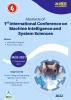# Prediction of Data Traffic Flow Using Machine Learning Approach

## Authors

Department of Computer Engineering, Faculty of Engineering & Technology, Jamia Millia Islamia, New Delhi, India
Ajay Kumar
Department of Computer Science & Informatics, Central University of Himachal Pradesh, Dharamshala

### Synopsis

Background: The aim of this problem is to present Prediction of Internet Data Traffic Flow in a university using Machine Learning to predict high accuracy. In the proposed model three different algorithms of Machine Learning (Gradient Boosting algorithm, Decision Tree and Random Forest) are used and compared among themselves with different parameter. It was found that Gradient Boosting gave the best result with respect to another model. In comparing the different Machine Learning algorithm, the training time and the accuracy of the test data is considered. Decision Tree gives the minimum accuracy of 84.20%, whereas 97.50% accuracy was given by Gradient Boosting.

Objective:  The main motive of this research is to predict the Internet data flow in the university through machine learning techniques to predict high accuracy of data flow. In this regard our proposed model has considered three different machine learning algorithms (Gradient Boosting algorithm, Decision Tree, Random Forest Regressor) has been used and compared with different parameters in order to achieve more accurate result in terms of predicting the data value. The researcher founded that among the three algorithm (Gradient Boosting algorithm, Decision Tree, Random Forest Regressor) Gradient Boosting has given the better result with maximum accuracy of 97.50% with respect to the Decision Tree which has least accuracy 84.20% and Random Forest Regressor with accuracy 94.10% other two algorithm

Methodology: The researcher explains the proposed method with all sorts of attributes involved in it. The variable which are selected, the independent variables have been discussed in data understanding section and the predicted Internet Traffic is the target dependent continuous variable. While predicting the daily Internet flow it was observed that, the flow gets effected due to various reason. Such as days of week – on Friday the internet consumption was seen more than normal days, on any events in the university like sports day, annual day etc. All these problems are carefully analyzed to overcome such issues.

Result and discussion: The researcher has used three performance indicator that are Root Mean Square Error (RMSE), Mean Square Error (MSE) and R2 score. The following quality metrics has been computed using the scikit learn application programming interface (API). The three indicators are defining as – MASE, RMSE, R2

Table 1: Accuracy comparison of the MAE, RMSE, R2 of model for GBR, DT and RFR

Machine Learning Algorithm

MAE

RMSE

R2

Accuracy

Decision Tree

0.4915

0.6098

0.8945

84.20%

0.3353

0.4253

0.9801

97.50%

Ensembele Method: Random Forest Regressor

1.0552

1.7013

0.9903

94.10%

Future Work: For Future work, anomalies detection can be added in the model of future traffic forecasting by using deep learning algorithm. Which can help in improving the security. Further different routing topologies can be added further to have a powerful prediction for improved performance of the model.Published
January 28, 2022